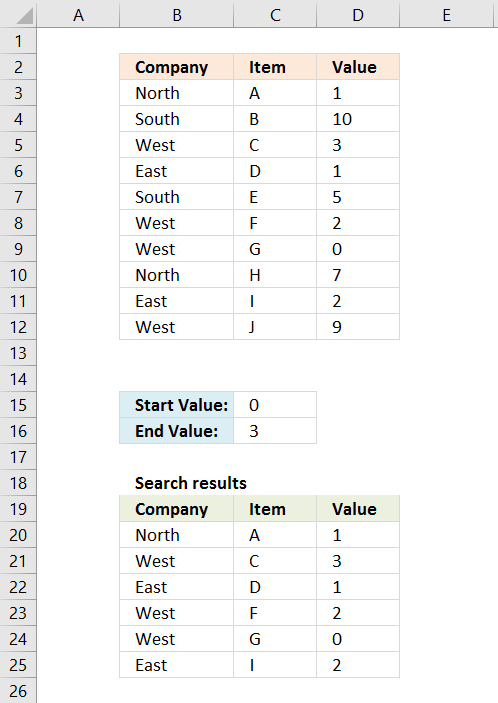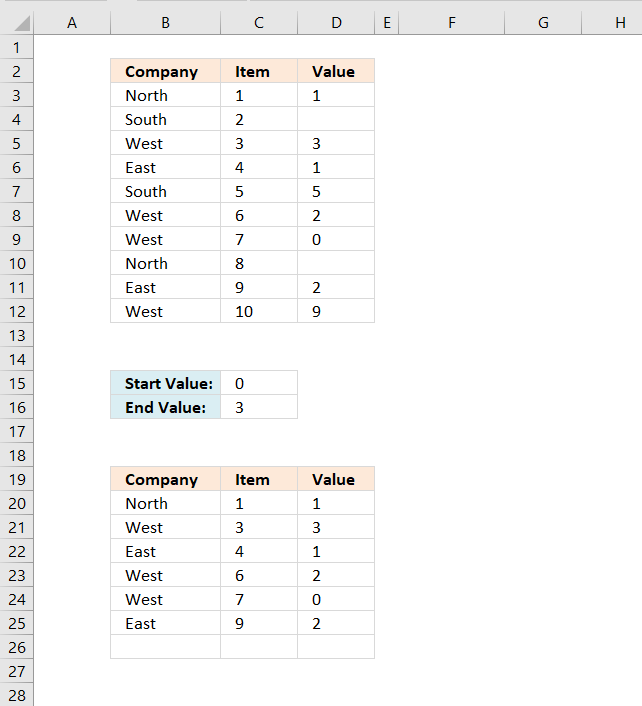Author: Oscar Cronquist Article last updated on May 08, 2019Question: I have a list and I want to filter out all rows that have a value (Column C) that is bigger or equal than zero and smaller or equal than three?

The picture above shows you the formula result in cell range B20:C25. The numerical search range is 0 to 3.

Array formula in B20:

=INDEX(\$B\$3:\$D\$12, SMALL(IF((\$C\$16>=\$D\$3:\$D\$12)*(\$C\$15<=\$D\$3:\$D\$12), MATCH(ROW(\$C\$3:\$C\$12), ROW(\$C\$3:\$C\$12))), ROWS(\$A\$1:A1)), COLUMN(A1))

To enter an array formula, type the formula in cell B20 then press and hold CTRL + SHIFT simultaneously, now press Enter once. Release all keys.

The formula bar now shows the formula with a beginning and ending curly bracket telling you that you entered the formula successfully. Don't enter the curly brackets yourself.

Copy cell B20 and paste to cell range B20:D25.

#### Watch a video where I explain the formula

https://youtu.be/9mGIsO3c3MA

### Explaining formula in cell B20

#### Step 1 - Check cell range D3:D12 if equal to or less than cell C16 and equal to or larger than C15

(\$C\$16>=\$D\$3:\$D\$12)*(\$C\$15<=\$D\$3:\$D\$12)

becomes

(3>={1;10;3;1;5;2;0;7;2;9})*(0<=\${1;10;3;1;5;2;0;7;2;9})

becomes

{TRUE;FALSE;TRUE;TRUE;FALSE;TRUE;TRUE;FALSE;TRUE;FALSE})*({TRUE;TRUE;TRUE;TRUE;TRUE;TRUE;TRUE;TRUE;TRUE;TRUE})

and returns

{1;0;1;1;0;1;1;0;1;0}

 Boolean Boolean Multiply Add FALSE FALSE 0  (zero) 0 (zero) FALSE TRUE 0  (zero) 1 TRUE TRUE 1 2

#### Step 2 - Convert array to row numbers

The IF function lets you use a logical expression to determine which value (argument) to return.

IF((\$C\$16>=\$D\$3:\$D\$12)*(\$C\$15<=\$D\$3:\$D\$12), MATCH(ROW(\$C\$3:\$C\$12), ROW(\$C\$3:\$C\$12)))

becomes

IF({1;0;1;1;0;1;1;0;1;0}, MATCH(ROW(\$C\$3:\$C\$12), ROW(\$C\$3:\$C\$12)))

becomes

IF({1;0;1;1;0;1;1;0;1;0}, {1;2;3;4;5;6;7;8;9;10})

and returns

{1;FALSE;3;4;FALSE;6;7;FALSE;9;FALSE}

#### Step 3 - Get k-th smallest row number

To be able to return a single value from the array we need to use the SMALL function to extract a single row number. The second argument in the SMALL function uses the ROWS function with an expanding cell reference to extract a new value in each cell.

SMALL(IF((\$C\$16>=\$D\$3:\$D\$12)*(\$C\$15<=\$D\$3:\$D\$12), MATCH(ROW(\$C\$3:\$C\$12), ROW(\$C\$3:\$C\$12))), ROWS(\$A\$1:A1))

becomes

SMALL({1;FALSE;3;4;FALSE;6;7;FALSE;9;FALSE}, ROWS(\$A\$1:A1))

becomes

SMALL({1;FALSE;3;4;FALSE;6;7;FALSE;9;FALSE}, 1)

and returns 1.

#### Step 4 - Return value based on row and column number

The INDEX function returns a value from a cell range based on a row and column number, our cell range is a single column so we need to only specify a row number in order to get the correct value.

INDEX(\$B\$3:\$D\$12, SMALL(IF((\$C\$16>=\$D\$3:\$D\$12)*(\$C\$15<=\$D\$3:\$D\$12), MATCH(ROW(\$C\$3:\$C\$12), ROW(\$C\$3:\$C\$12))), ROWS(\$A\$1:A1)), COLUMN(A1))

becomes

INDEX(\$B\$3:\$D\$12, 1, COLUMN(A1))

becomes

INDEX(\$B\$3:\$D\$12, 1, 1)

and returns "North" in cell B20.

### Exclude blank valuesThe image above shows some records contain a blank value, the following formula ignores records that contain a blank value.

Formula in cell B20:

=INDEX(\$B\$3:\$D\$12, SMALL(IF((\$C\$16>=\$D\$3:\$D\$12)*(\$C\$15<=\$D\$3:\$D\$12)*(\$D\$3:\$D\$12<>""), MATCH(ROW(\$C\$3:\$C\$12), ROW(\$C\$3:\$C\$12))), ROWS(\$A\$1:A1)), COLUMNS(\$A\$1:A1))